Examples from NCERT Book

Class 10
Chapter 10 Class 10 - Light - Reflection and Refraction

## An object, 4.0 cm in size, is placed at 25.0 cm in front of a concave mirror of focal length 15.0 cm. At what distance from the mirror should a screen be placed in order to obtain a sharp image?  Find the nature and the size of the image.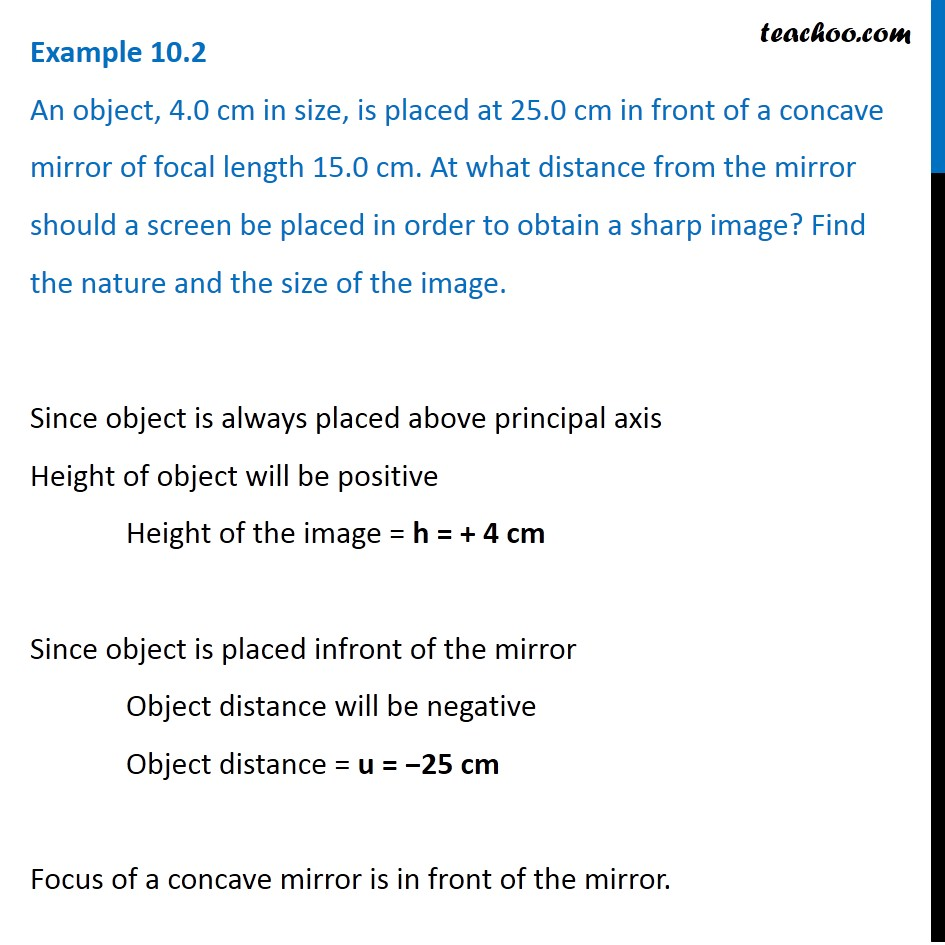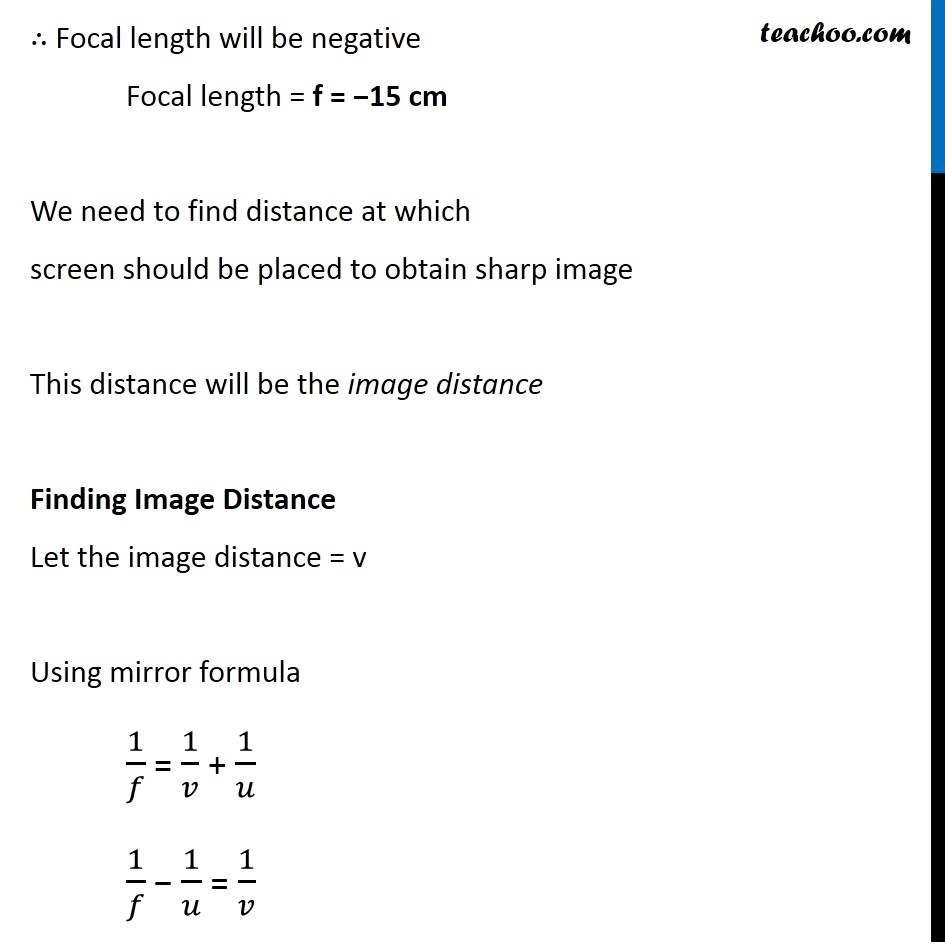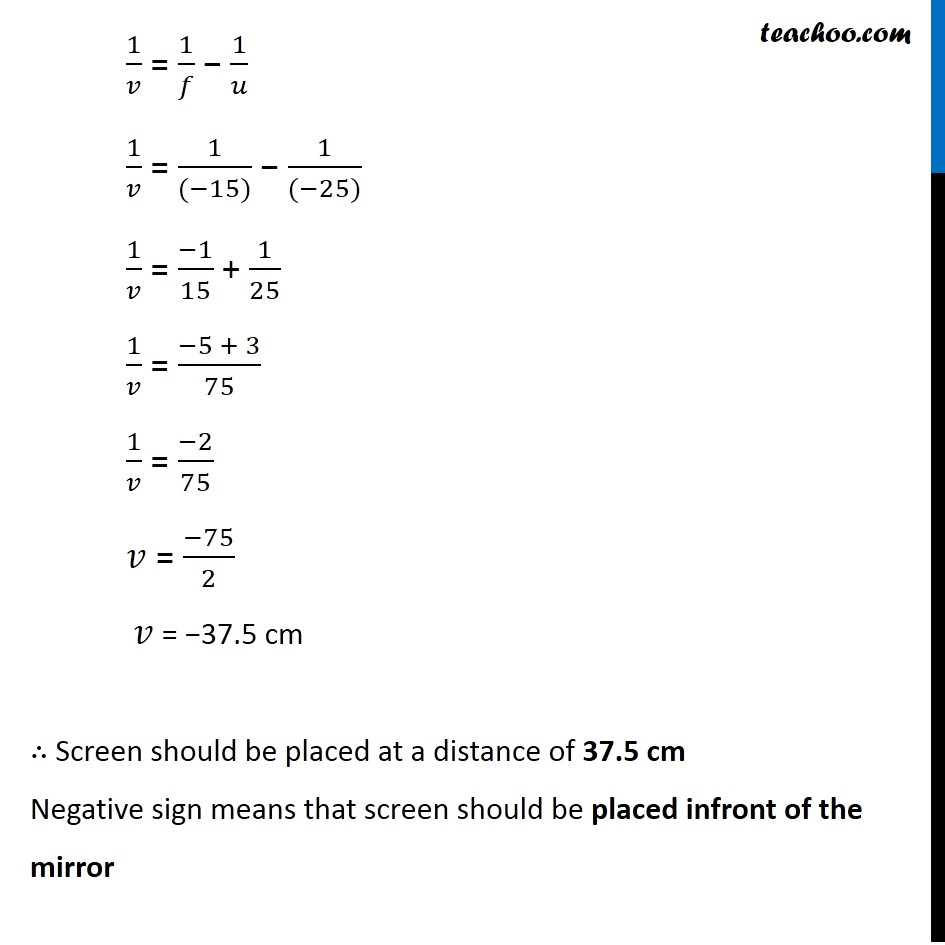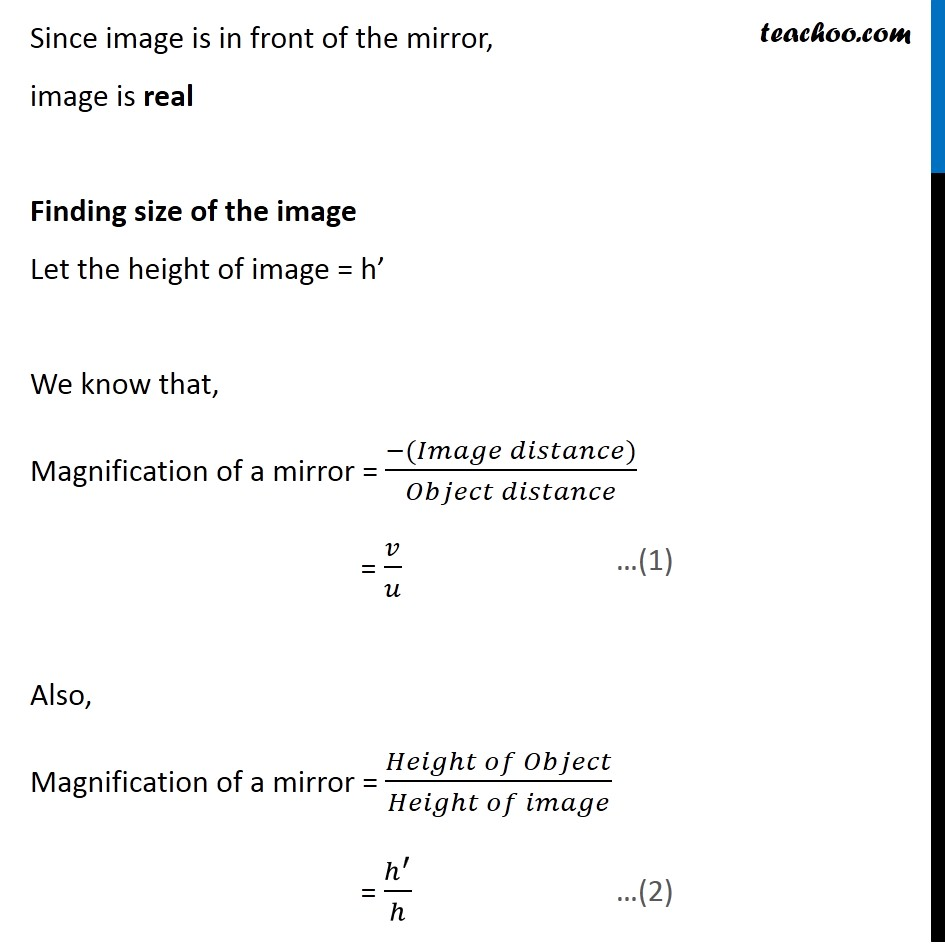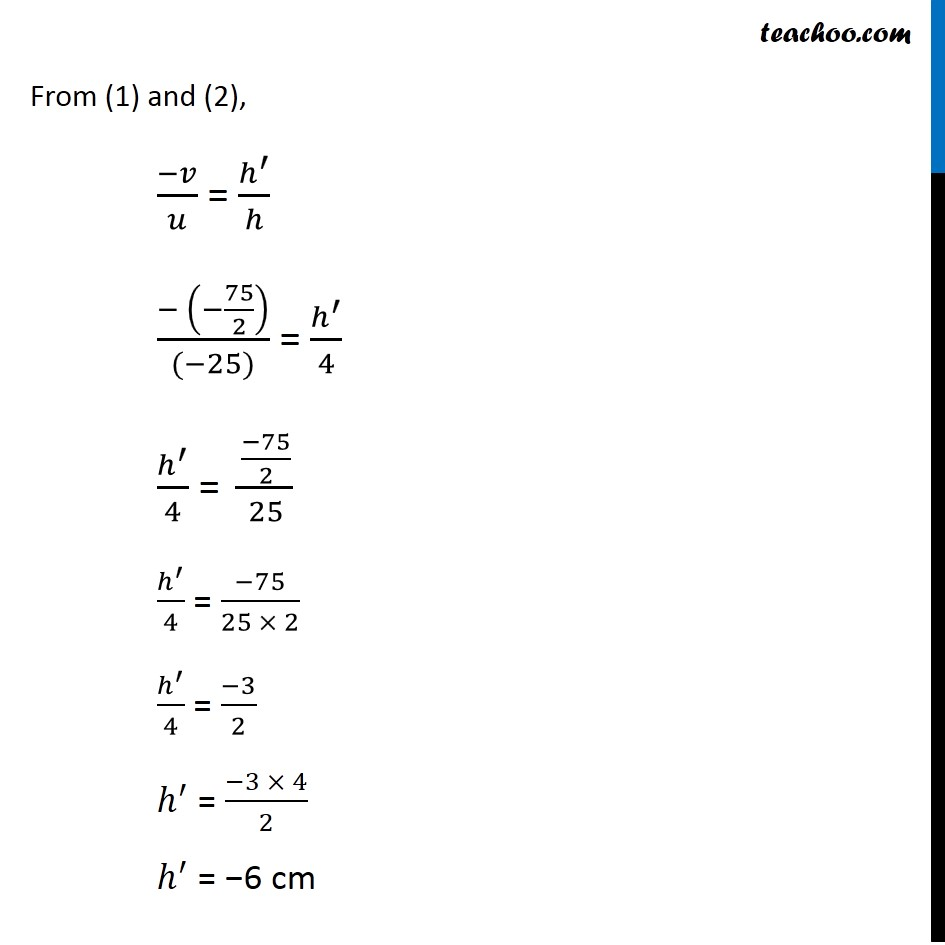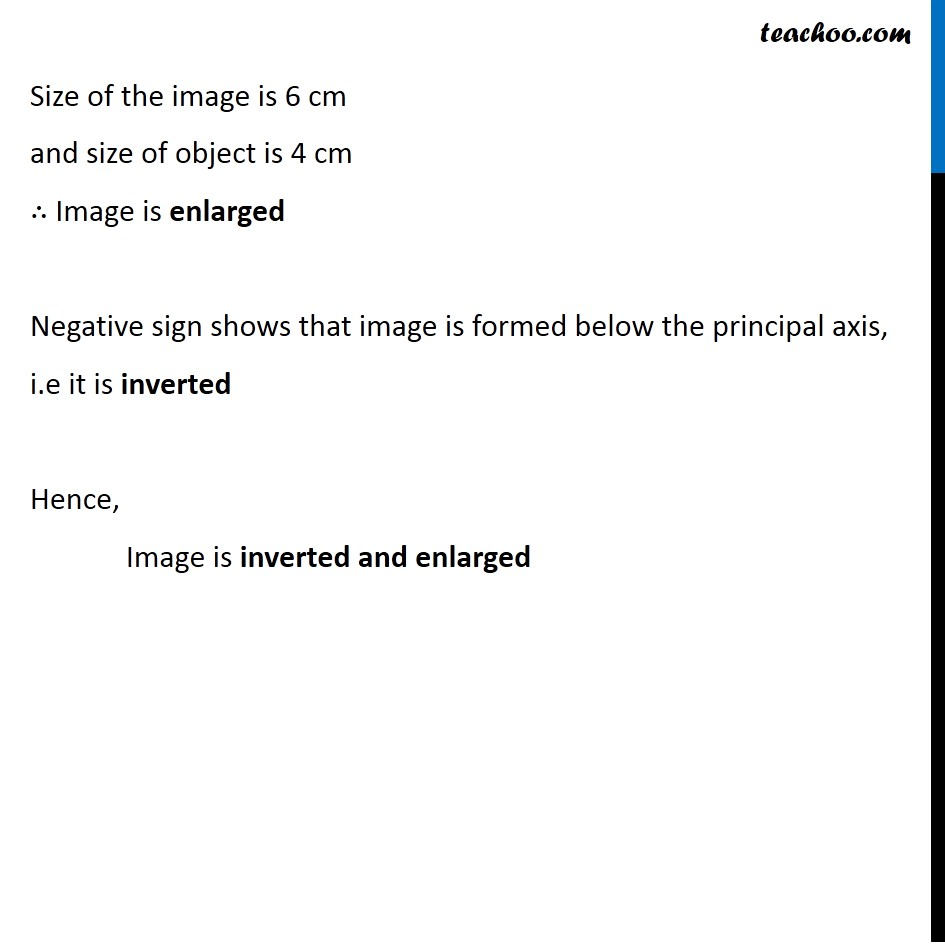Learn in your speed, with individual attention - Teachoo Maths 1-on-1 Class

### Transcript

Example 10.2 An object, 4.0 cm in size, is placed at 25.0 cm in front of a concave mirror of focal length 15.0 cm. At what distance from the mirror should a screen be placed in order to obtain a sharp image? Find the nature and the size of the image. Since object is always placed above principal axis Height of object will be positive Height of the image = h = + 4 cm Since object is placed infront of the mirror Object distance will be negative Object distance = u = −25 cm Focus of a concave mirror is in front of the mirror. ∴ Focal length will be negative Focal length = f = −15 cm We need to find distance at which screen should be placed to obtain sharp image This distance will be the image distance Finding Image Distance Let the image distance = v Using mirror formula 1/𝑓 = 1/𝑣 + 1/𝑢 1/𝑓 − 1/𝑢 = 1/𝑣 1/𝑣 = 1/𝑓 − 1/𝑢 1/𝑣 = 1/((−15)) − 1/((−25)) 1/𝑣 = (−1)/15 + 1/25 1/𝑣 = (−5 + 3)/75 1/𝑣 = (−2)/75 𝑣 = (−75)/2 𝑣 = −37.5 cm ∴ Screen should be placed at a distance of 37.5 cm Negative sign means that screen should be placed infront of the mirror Since image is in front of the mirror, image is real Finding size of the image Let the height of image = h’ We know that, Magnification of a mirror = (−(𝐼𝑚𝑎𝑔𝑒 𝑑𝑖𝑠𝑡𝑎𝑛𝑐𝑒))/(𝑂𝑏𝑗𝑒𝑐𝑡 𝑑𝑖𝑠𝑡𝑎𝑛𝑐𝑒) = 𝑣/𝑢 Also, Magnification of a mirror = (𝐻𝑒𝑖𝑔ℎ𝑡 𝑜𝑓 𝑂𝑏𝑗𝑒𝑐𝑡)/(𝐻𝑒𝑖𝑔ℎ𝑡 𝑜𝑓 𝑖𝑚𝑎𝑔𝑒) = ℎ^′/ℎ From (1) and (2), (−𝑣)/𝑢 = ℎ^′/ℎ (− (−75/2))/((−25)) = ℎ^′/4 ℎ^′/4 = ((−75)/2)/25 ℎ^′/4 = (−75)/(25 × 2) ℎ^′/4 = (−3)/2 ℎ^′ = (−3 × 4)/2 ℎ^′ = −6 cm Size of the image is 6 cm and size of object is 4 cm ∴ Image is enlarged Negative sign shows that image is formed below the principal axis, i.e it is inverted Hence, Image is inverted and enlarged